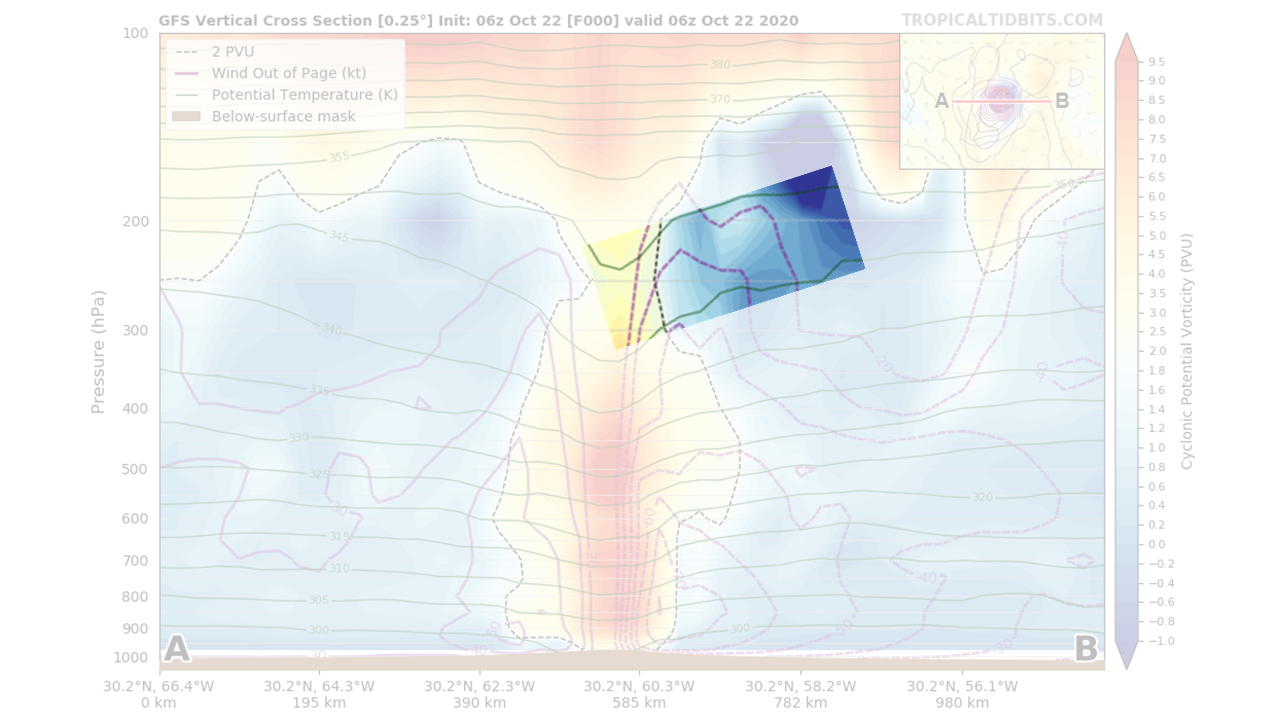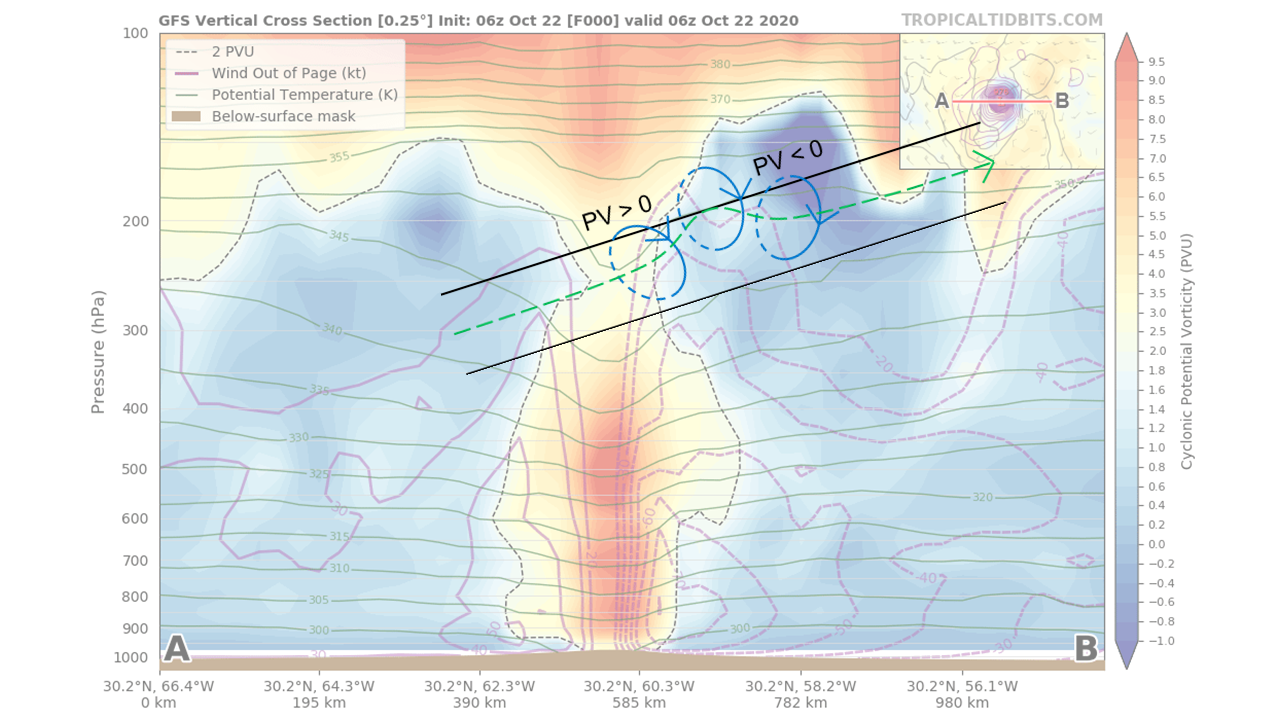# The Role of Diabatic Vorticity Tilting in PV Distribution in the Outflow Layer of Hurricane Epsilon (2020)

Published:This post explains why diabatic vorticity tilting plays an essential role in the distribution of potential vorticity (PV) in the outflow layer of the hurricane. In the zonal vertical cross section through the center of Hurricane Epsilon (2020) above, I highlight the region from the center to 300 km toward the east between 345 K and 350 K isentropes. Why is PV near the center so strongly cyclonic (> 2 PVU)? Why is PV on the skirt anticyclonic (< 0 PVU)?

For the strongly cyclonic PV near the center, vertical advection of PV seems an appealing explanation. Indeed, it is positive owing to the upward motion and the vertically decreasing PV. However, such advection cannot conserve PV because it is cross-isentropic (i.e., diabatic), and diabatic vorticity squeezing due to vertically decreasing heating rate is a negative effect here. Nonetheless, diabatic vorticity tilting is positive here. These contributors of different signs complicate the qualitative PV analysis. Fortunately, Haynes and McIntyre (1990) gave insight to eliminate some contributors. They proved that the net effect of all cross-isentropic PV density fluxes is displacement of the isentropes (scientific statements cannot be proved, but this is a provable mathematical consequence of how PV is defined). Hence, cross-isentropic PV density fluxes are dynamically insignificant because they offset one another leaving no net flux through the isentropes.

Focusing on small Rossby number, Haynes and McIntyre (1990) explained that diabatic heating changes PV through concentration and dilution. While PV density cannot permeate an isentropic level, diabatic heating transports mass from a lower isentropic layer to higher, concentrating the PV below the heating maximum and diluting above. In terms of PV density flux, the dilution and concentration are associated with divergence and convergence of advective flux along the isentropes. This conceptual model predicts that PV in the outflow layer of a hurricane should be diluted and thus anomalously weak and still cyclonic. However, this is not observed.

My paper (Ong 2020) gives a proper conceptual model considering the diabatic flux along the isentropes derived by Haynes and McIntyre (1990) but ignored for small Rossby number. In short, diabatic heating transports momentum from a lower isentropic layer to higher, which yields a PV density flux opposing the along-isentropic vorticity vector. To illustrate, I annotate the cross section as below. With respect to the hurricane center, given the radially outward vorticity, diabatic heating fluxes PV radially inward, which explains both the strongly cyclonic PV near the center and the anticyclonic PV on the skirt. As explained in Ong 2020, the diabatic flux along the isentropes is equivalent to diabatic tilting of vorticity from along-isentropic to cross-isentropic. Therefore, the diabatic tilting is essential for creating the radially inward PV gradient in the outflow layer of the hurricane. This may explain the symmetric instability (i.e., anticyclonic PV) in the outflow layer of Hurricane Ivan (2004) found by Molinari and Vollaro (2014).All graphics in this blog post are based on a vertical cross section created at tropicaltidbits.com

Related research project: diabatic tilting of potential vorticity

Tags: